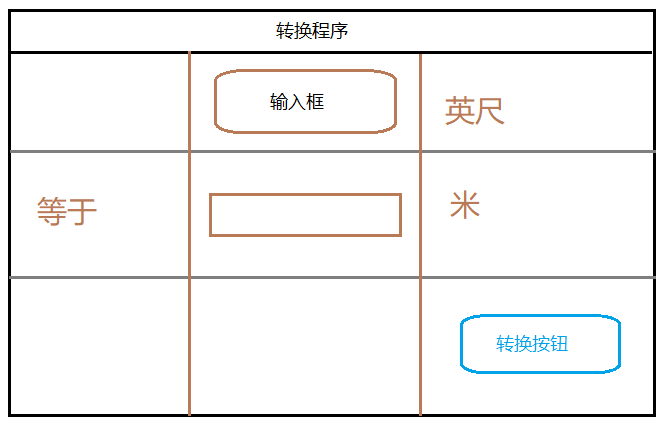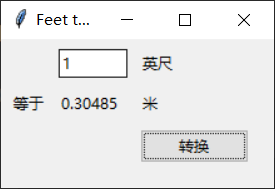# 你的第一个 GUI 程序——Tkinter教程系列02

Tk 系列教程：

## 设计## 代码

from tkinter import *
from tkinter import ttk

def calculate(*args):
try:
value = float(feet.get())
meters.set((0.3048 * value * 10000.0 + 0.5)/10000.0)
except ValueError:
pass

root = Tk()
root.title("Feet to Meters")
mainframe = ttk.Frame(root, padding="3 3 15 15")
mainframe.grid(column=0, row=0, sticky=(N, W, E, S))
mainframe.columnconfigure(0, weight=1)
mainframe.rowconfigure(0, weight=1)

feet = StringVar()
meters = StringVar()

feet_entry = ttk.Entry(mainframe, width=7, textvariable=feet)
feet_entry.grid(column=2, row=1, sticky=(W, E))

ttk.Label(mainframe, textvariable=meters).grid(column=2, row=2, sticky=(W, E))
ttk.Button(mainframe, text="转换", command=calculate).grid(column=3, row=3, sticky=W)

ttk.Label(mainframe, text="英尺").grid(column=3, row=1, sticky=W)
ttk.Label(mainframe, text="等于").grid(column=1, row=2, sticky=E)
ttk.Label(mainframe, text="米").grid(column=3, row=2, sticky=W)

feet_entry.focus()
root.bind('<Return>', calculate)

root.mainloop()## 逐步解释

from tkinter import *
from tkinter import ttk


root = Tk()
root.title("Feet to Meters")
mainframe = ttk.Frame(root, padding="3 3 15 15")
mainframe.grid(column=0, row=0, sticky=(N, W, E, S))
mainframe.columnconfigure(0, weight=1)
mainframe.rowconfigure(0, weight=1)


calculate函数将会在后面讲解，之所以放到前面，是因为后面的许多语句需要调用它。

root = Tk() 语句构建了一个main窗口，也被称为root窗口。使用 root.title("title") 为窗口赋予一个名字。ttk.Frame(root, padding="3 3 15 15") 建立一个框架，这个框架分为三行三列，像素为15。我们将这个框架放置到到root窗口中，不同的是，我们的所有组件都被放到了这个框架中而不是root窗口。

columncoonfigurerowconfigure 告诉 Tk， 当主窗口重新改变了大小，那么在这之上的 Frame 框架也应该变化，以占用多余的空间。

feet = StringVar()
meters = StringVar()
feet_entry = ttk.Entry(mainframe, width=7, textvariable=feet)
feet_entry.grid(column=2, row=1, sticky=(W, E))

ttk.Label(mainframe, textvariable=meters).grid(column=2, row=2, sticky=(W, E))
ttk.Button(mainframe, text="转换", command=calculate).grid(column=3, row=3, sticky=W)


1. 创建
2. 放置

ttk.Label(mainframe, text="英尺").grid(column=3, row=1, sticky=W)
ttk.Label(mainframe, text="等于").grid(column=1, row=2, sticky=E)
ttk.Label(mainframe, text="米").grid(column=3, row=2, sticky=W)


for child in mainframe.winfo_children():
feet_entry.focus()
root.bind('<Return>', calculate)


def calculate(*args):
try:
value = float(feet.get())
meters.set((0.3048 * value * 10000.0 + 0.5)/10000.0)
except ValueError:
pass


feet = StringVar()
meters = StringVar()
feet_entry = ttk.Entry(mainframe, width=7, textvariable=feet)
ttk.Label(mainframe, textvariable=meters).grid(column=2, row=2, sticky=(W, E))

root.mainloop()


## 后记

Stay Hungry, Stay Folish.
posted @ 2021-04-10 01:02  RelaxCN  阅读(490)  评论(2编辑  收藏  举报